# Previous Year Question Answers: Motion - Science Class 9

Q.1. The brakes applied to a car produce an acceleration of 6 ms-2 in the opposite direction to the motion. If the car takes 2 s to stop after the application of brakes, calculate the distance it travels during this time.    [CBSE 2016]
Ans.
Acceleration, a = - 6 ms-2
Final velocity, v = 0
Time, t = 2 s
Initial velocity, u = v - at = 0 - (-6) (2)
= 12 ms-1
Distance travelled,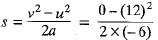= 12 m

Q.2. The graph given below shows the position of a body at different times. Calculate the speed of the body as it moves from:    [CBSE 2016]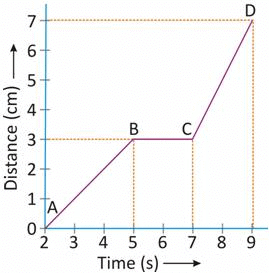(i) A to B
(ii) B to C
(iii) C to D
Ans.
(i) Speed from A to B = Slope AB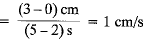(ii) Speed from B to C = slope BC = zero
(iii) Speed from C to D = slope CD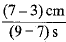= 2 cm/s

Q.3. (a) A train 100 m along is moving with a constant velocity of 60 kmh-1. Find the time it takes to cross the bridge 1 km long.
(b) The slope of the line on a position-time graph reveals information about an object’s velocity. What conclusion can you draw regarding the motion of an object, if the position-time graph is a:
(i) horizontal line parallel to time axis.
(ii) straight line at 45° to time axis.
(iii) curve    [CBSE 2016]
Ans.
(a) Distance travelled by train = length of bridge + length of train = 1000 m + 100 m = 1100 m
v = 60 km/h =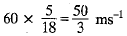Time taken,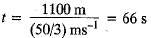(b) (i) The object is at rest
(ii) The object is moving at constant velocity.
(iii) The motion of the object is non- uniform.

Q.4. Name a device that measures distance travelled by automobiles. A body travels a distance of 15 m from A to B and then moves a distance of 20 m at right angle to AB. Calculate the total distance travelled and the displacement.    [CBSE 2016]
Ans.
An odometer measures the distance travelled in automobiles.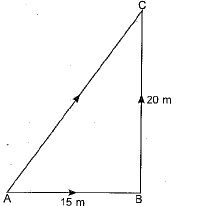Let body moves 20 m from B to C.
Distance = AB + BC
= 15 m + 20 m = 35 m
Displacement =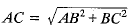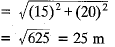Q.5. A powerful motorcycle can accelerate from rest to 28 m/s in only 4s.    [CBSE 2016]
(a) What is its average acceleration?
(b) How far does it travel in that time?
Ans.
Initial velocity, u = 0, final velocity v = 28 ms-1, time t = 4 s.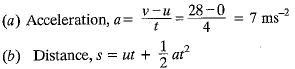Q.6. What type of motion is described by a stone which moves in a circular path with constant speed?    [CBSE 2016]
Ans.
Uniform circular motion.

Q.7.  A car travels a distance of 360 km in 5 hours. What is the speed in ms-1?    [CBSE 2016]
Ans.
Distance = 360 km = 360 x 10m;
Time 5 h = 5 x 3600 s
Speed =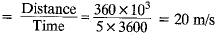Q.8. An electric train is moving with a velocity of 30 m/s. How much distance will it travel in 30 S?    [CBSE 2016]
Ans.
Velocity, v = 30 ms-1; time, t = 30 s
Distance = vt = 30 x 30 = 900 m

Q.9. Starting from a stationary position, Anil paddles his bicycle to attain a velocity of 10 m s-1 in 25 s. Then, he applies brakes such that he again comes to rest after next 50 s. Calculate the acceleration of the bicycle in both cases. Also, find the total distance covered by Anil.    [CBSE 2011, 2014, 2016]
Ans.
In first case initial velocity u1 = 0, time t1 = 25 s and final velocity v= 10 m s-1
∴ acceleration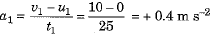and distance covered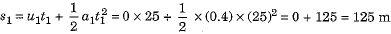After applying the brakes in second case initial velocity u2 = 10 m s-1, time t2 = 50 s and final velocity v2 = 0
∴ acceleration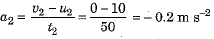and distance covered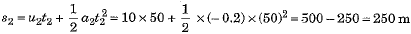Total distance covered by Anil s = s1 + s2 = 125 + 250 = 375 m

Q.10. Starting from rest, Rohan pressed the accelerator of his car to attain a velocity of 12 m s-1 in 30 s. Then he applies brakes such that the velocity of the car comes down to 8 m s-1 in the next 5 s. Calculate the acceleration of the car in both the cases.    [CBSE 2016]
Ans.
For first part of motion, initial velocity u = 0, final velocity v = 12 m s-1 and time t = 30 s.
∴ acceleration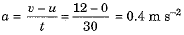For subsequent motion, initial velocity u' = 12 m s-1, final velocity v' = 8 m s-1 and time t' = 5s.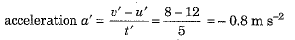Q.11. Name the physical quantities denoted by:
(a) the slope of the distance-time graph.
(b) the area under velocity-time graph.
(c) the slope of the velocity-time graph.    [CBSE 2013, 2016]
Ans.
(a) In general, the slope of the distance-time graph gives the speed of object. However, for motion along a straight path in a given direction slope of the distance-time graph gives the magnitude of velocity.
(b) The area under velocity-time graph gives the magnitude of displacement (or the distance) covered by the object.
(c) The slope of velocity-time graph gives the acceleration of the moving object.

Q.12. What do you mean by uniform and non-uniform motion? What can be the shape of the path covered by a moving object to have (a) uniform speed and (b) uniform velocity?    [CBSE 2015, 2016]
Ans.
The motion of an object is said to be uniform, if it covers equal displacements m equal intervals of time.
The motion of an object is said to be non-uniform if it covers unequal displacements in equal intervals of time or equal displacements in unequal intervals of time.
(a) If a moving object is to have a uniform speed then the shape of the path covered by it can be either a straight line or a curve.
(b) If a moving object is to have uniform velocity then its path must be a straight line path along a given direction.

Q.13. Name the physical quantity whose SI urnis are as given below:
(i) m/s
(ii) m/s2    [CBSE 2016]
Ans.
(i) Speed and velocity (ii) accelertion.

Q.14. A train starting from a railway station attains a velocity of 30 m/s in one minute. What is its acceleration?    [CBSE 2016]
Ans.
Here initial velocity u = 0, final velocity v = 30 m/s and time t = 1 minute = 60 s.
∴ Acceleration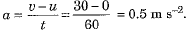Q.15. Name the device that is fitted in automobiles to show the distance travelled by them.    [CBSE 2016]
Ans.
An odometer measures the distance travelled by automobile.

Q.16. Name the physical quantity that essentially varies as a body moves.    [CBSE 2016]
Ans.
Position of the body.

Q.17. A motorcyclist riding motorcycle A, who is travelling at 36 km h-1, applies the brakes and stops the motorcycle in 10 s. Another motorcyclist of motorcycle B, who is travelling at 18 km h-1, applies the brakes and stops the motorcycle in 20 s. Plot speed-time graphs for the two motorcycles. Which of the two motorcycles travelled farther before it came to a stop?    [CBSE 2011, 2014, 2015]
Ans.
For motorcycle A :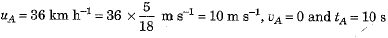For motorcycle B :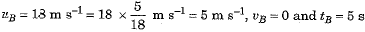Speed-time graphs for motorcycles A arid B have been plotted in Fig. 8.27.
Distance covered by motorcycle A
sA = area OPQ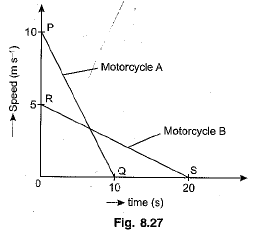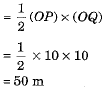and distance covered by motorcycle B
sB = area ORS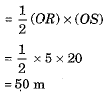Thus, both motorcycles travelled equal distances before coming to a stop.

(i) State the type of motion shown by a freely falling stone.
(ii) When a stone is thrown vertically upwards its velocity is continuously decreases. Why?
(iii) Give an example of a motion in which average velocity is zero, but the average speed is not zero.    [CBSE 2015]
Ans.
(i) Motion of a freely falling stone is an uniformly accelerated motion.
(ii) When a stone is thrown vertically upwards, a constant acceleration due to gravity acts on it in the downward direction. It causes a retardation. So, velocity of stone continuously goes on decreasing.
(iii) Let a person goes from station A to station B and then returns back to station A covering a finite distance in certain time. Here average speed of person is finite. However, his average velocity is zero because there is no net displacement of the person.

Q.19. Name the type of motion in which a body has uniform speed but not uniform velocity?    [CBSE 2010, 2015]
Ans.
Uniform circular motion.

Q.20. What is the numerical ratio of average velocity to average speed of an object when it is moving along a straight path?    [CBSE 2013, 2015]
Ans.
For motion along a straight path in a given direction the magnitude of average velocity is same as average speed and hence the numerical ratio is 1.

Q.1. The speed-time graph of a body is as shown in Fig. 8.34: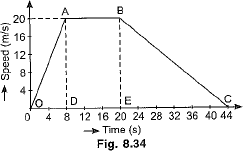(a) What type of motion is represented by OA?
(b) What type of motion is represented by AB?
(c) Calculate the retardation of the body.
(d) Calculate the distance travelled by the body from O to C.
(e) What is the average velocity of the body for its entire journey?    [CBSE 2016]
Ans. (a) OA represents uniformly accelerated motion.
(b) AB represents uniform motion without any acceleration.
(c) BC represents the retarded motion, in which magnitude of retardation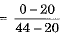(d) Distance travelled by the body from O to C, s = area of the trapezium OABC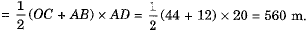(e) Average velocity of the body for entire journey =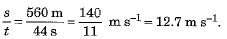Q.2. An object starts linear motion with a velocity u and under uniform acceleration a it acquires a velocity v in time t. Draw velocity-time graph. From this graph obtain the following equations:     [CBSE 2015, 2016]
(a) v = u + at,
(b) s = ut + 1/2 at2.
Ans.
The velocity-time graph has been shown in Fig. 8.30.
At t = 0, the initial velocity is u (at point A) and then increases to v (at point B) in time t.
(a) Draw perpendicular lines BC and BE from point B on the time and velocity axes respectively, so that OA = u, OE =BC = v.
Also draw a line AD parallel to time axis, so that OC = AD = t.
Then change in velocity in time t = BC - OA = BC - CD = BD
But BC = v and CD = OA = u. Hence, BD = v - u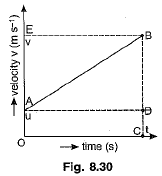From the velocity-time graph, the acceleration of the object is given by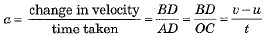∴ at = v - u ⇒ v = u + at
(b) According to velocity-time graph the total distance covered by the object is obtained by the area under the graph.
Hence, distance s travelled by the object = area of the trapezium OABC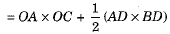But OA = u, OC = t, AD = OC = t and BD = change in velocity = v - u = at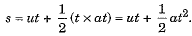Q.3. From the velocity-time graph of uniformly accelerated motion, establish the equation 2as = v- u2 (equation for position-velocity relation).    [CBSE 2011, 2012, 2015]
Ans.
As shown in Fig. 8.30, the distance s travelled by the object in time t is given by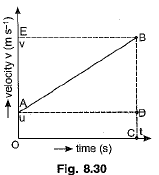s = area of the trapezium OABC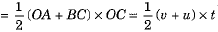...(i)
From velocity-time relation v = u + at, we have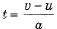...(ii)
Substituting value of time t from equation (ii) into (i), we get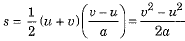⇒ 2as = v2 - u2    ...(iii)
which is the requisite equation.

Q.4. The velocity-time graph of an object is as shown below: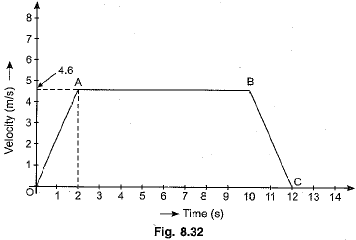(а) Identify the kind of motion of the object represented by lines OA and BC.
(b) With what velocity the object is moving at t = 8 seconds?
(c) Calculate the acceleration of the object in the following cases:
(i) Between the third and tenth second.
(ii) During the last two seconds.    [CBSE 2015]
Ans.
(a) The line OA represents uniformly accelerated motion. The line BC represents uniformly retarded motion.
(b) At time t = 8 s, the object is moving with a velocity of 4.6 m s-1.
(c) (i) Between 3rd and 10th second, object is moving with a constant velocity of 4.6 m s-1. Hence acceleration of object is zero.
(ii) During the last two seconds, velocity of object falls from u = 4.6 m s-1 to v = 0. Hence,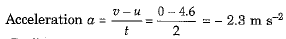The document Previous Year Question Answers: Motion | Science Class 9 is a part of the Class 9 Course Science Class 9.
All you need of Class 9 at this link: Class 9

## Science Class 9

66 videos|355 docs|97 tests

## FAQs on Previous Year Question Answers: Motion - Science Class 9

 1. What is motion?Ans. Motion is the change in position of an object with respect to its surroundings over time. It can be described in terms of distance, displacement, speed, velocity, and acceleration.
 2. What are the different types of motion?Ans. There are three main types of motion: linear motion, circular motion, and oscillatory motion. Linear motion refers to the movement along a straight line, circular motion involves movement in a circular path, and oscillatory motion is the back-and-forth movement of an object.
 3. What is the difference between speed and velocity?Ans. Speed is a scalar quantity that measures how fast an object is moving, while velocity is a vector quantity that includes both speed and direction. In other words, velocity specifies the speed and direction of an object's motion.
 4. What is the equation for calculating average speed?Ans. Average speed is calculated by dividing the total distance traveled by an object by the total time taken. The equation for average speed is: average speed = total distance / total time.
 5. What is acceleration?Ans. Acceleration is the rate at which an object's velocity changes over time. It can be calculated by dividing the change in velocity by the time taken. Acceleration can be positive (speeding up), negative (slowing down), or zero (constant velocity).

## Science Class 9

66 videos|355 docs|97 testsExplore Courses for Class 9 examSignup to see your scores go up within 7 days! Learn & Practice with 1000+ FREE Notes, Videos & Tests.
10M+ students study on EduRev
Track your progress, build streaks, highlight & save important lessons and more!
Related Searches

,

,

,

,

,

,

,

,

,

,

,

,

,

,

,

,

,

,

,

,

,

;